Spirals
 To the Main Page       "Mathematische Basteleien"

What is a spiral?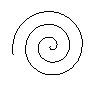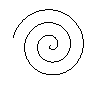A spiral is a curve in the plane or in the space, which runs around a centre in a special way.

Different spirals follow. Most of them are produced by formulas.

Spirals by Polar Equations top

Archimedean Spiral top
You can make a spiral by two motions of a point: There is a uniform motion in a fixed direction and a motion in a circle with constant speed. Both motions start at the same point.
 ...........................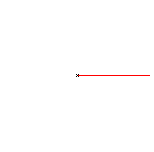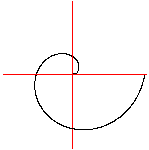(1) The uniform motion on the left moves a point to the right. - There are nine snapshots.
(2) The motion with a constant angular velocity moves the point on a spiral at the same time. - There is a point every 8th turn.
(3) A spiral as a curve comes, if you draw the point at every turn.

You get formulas analogic to the circle equations.
Circle
 ...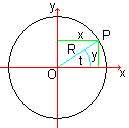... Let P be a point of a circle with the radius R, which is given by an equation in the centre position. There are three essential descriptions of the circle: (1) Central equation: x²+y² = R² or [y = sqr(R²-x²) und y = -sqr(R²-x²)], (2) Parameter form: x(t) = R cos(t), y(t) = R sin(t), (3) Polar equation: r(t) = R.
You give a point by a pair (radius OP, angle t) in the (simple) polar equation. The radius is the distance of the point from the origin (0|0). The angle lies between the radius and the positive x-axis, its vertex in the origin.

Spiral
The radius r(t) and the angle t are proportional for the simpliest spiral, the spiral of Archimedes. Therefore the equation is:
(3) Polar equation: r(t) = at [a is constant].
From this follows
(2) Parameter form:  x(t) = at cos(t), y(t) = at sin(t),
(1) Central equation:  x²+y² = a²[arc tan (y/x)]².

 ...... The Archimedean spiral starts in the origin and makes a curve with three rounds. The distances between the spiral branches are the same.  More exact: The distances of intersection points along a line through the origin are the same.

 ...... If you reflect an Archimedean spiral on a straight line, you get a new spiral with the opposite direction.  Both spirals go outwards. If you look at the spirals, the left one forms a curve going to the left, the right one forms a curve going to the right.
If you connect both spirals by a straight (red) or a bowed curve, a double spiral develops.

Equiangular Spiral (Logarithmic Spiral, Bernoulli's Spiral)     top
 ...... (1) Polar equation:  r(t) = exp(t).  (2) Parameter form: x(t) = exp(t) cos(t), y(t) = exp(t) sin(t).  (3) Central equation:  y = x tan[ln(sqr(x²+y²))].  The logarithmic spiral also goes outwards.  The spiral has a characteristic feature: Each line starting in the origin (red) cuts the spiral with the same angle.

More Spirals  top
If you replace the term r(t)=at of the Archimedean spiral by other terms, you get a number of new spirals. There are six spirals, which you can describe with the functions f(x)=x^a [a=2,1/2,-1/2,-1] and  f(x)=exp(x), f(x)=ln(x). You distinguish two groups depending on how the parameter t grows from 0.
 ...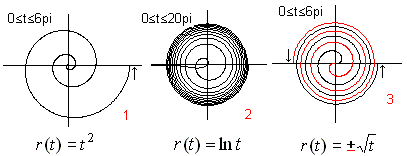......... If the absolute modulus of a function r(t) is increasing, the spirals run from inside to outside and go above all limits.  The spiral 1 is called parabolic spiral or Fermat's spiral.

 ...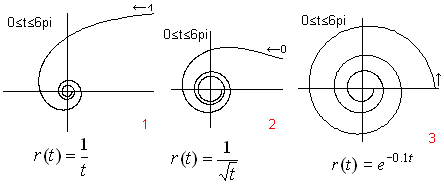.... If the absolute modulus of a function r(t) is decreasing, the spirals run from outside to inside. They generally run to the centre, but they don't reach it. There is a pole.  Spiral 2 is called the Lituus (crooked staff).
I chose equations for the different spiral formulas suitable for plotting.

Clothoide (Cornu Spiral)top
 ...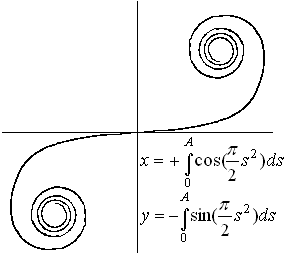.... The clothoid or double spiral is a curve, whose curvature grows with the distance from the origin. The radius of curvature is opposite proportional to its arc measured from the origin. The parameter form consists of  two equations with Fresnel's integrals, which can only be solved approximately. You use the Cornu spiral to describe the energy distribution of Fresnel's diffraction at a single slit in the wave theory.

Half circle spirals
 ...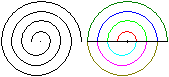... You can add half circles growing step by step to get spirals.  The radii have the ratios 1 : 1.5 : 2 : 2.5 : 3.....

Fibonacci Spiral
 ...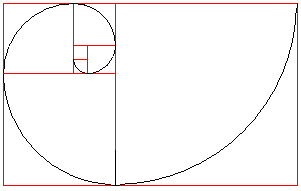... Draw two small squares on top of each other. Add a sequence of growing squares counter clockwise.  Draw quarter circles inside the squares (black). They form the Fibonacci Spiral.
The Fibonacci spiral is called after its numbers. If you take the length of the square sides in the order, you get the sequence 1,1,2,3,5,8,13,21, ... These are the Fibonacci numbers, which you can find by the recursive formula a(n)=a(n-1)+a(n-2) with [a(1)=1, a(2)=1, n>2].

Spirals Made of Line Segments    top
 ...... The spiral is made by line segments with the lengths 1,1,2,2,3,3,4,4,....  Lines meet one another at right angles.

 ...... Draw a spiral in a crossing with four intersecting straight lines, which form 45° angles. Start with the horizontal line 1 and bend the next line perpendicularly to the straight line. The line segments form a geometric sequence with the common ratio sqr(2).  If you draw a spiral into a straight line bundle, you approach the logarithmic spiral, if the angles become smaller and smaller.

 ...... The next spiral is formed by a chain of right angled triangles, which have a common side. The hypotenuse of one triangle becomes the leg of the next. First link is a 1-1-sqr(2)-triangle.  The free legs form the spiral. It is special that the triangles touch in line segments. Their lengths are the roots of the natural numbers. You can proof this with the Pythagorean theorem.  This figure is called root spiral or root snail or wheel of Theodorus.

 ...... Squares are turned around their centre with 10° and compressed at the same time, so that their corners stay at the sides of their preceding square.  Result: The corners form four spiral arms. The spiral is similar to the logarithm spiral, if  the angles get smaller and smaller. You can also turn other regular polygons e.g. an equilateral triangle. You get similar figures.
This picture reminds me of the programming language LOGO of the early days of computing (C64-nostalgia).

Three-dimensional Spirals top
Helix
 ...... If you draw a circle with x=cos(t) and y=sin(t) and pull it evenly in z-direction, you get a spatial spiral called cylindrical spiral or helix.

The picture pair makes a 3D view possible.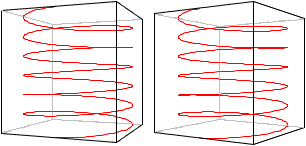...... Reflect the 3D-spiral on a vertical plane. You get a new spiral (red) with the opposite direction.  If you hold your right hand around the right spiral and if your thumb points in direction of the spiral axis, the spiral runs clockwise upward. It is right circular.  You must use your left hand for the left spiral. It is left circular. The rotation is counter clockwise.  Example: Nearly all screws have a clockwise rotation, because most of the people are right-handed.

 ...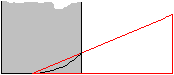... In the "technical" literature the right circular spiral is explained as follows: You wind a right- angled triangle around a cylinder. A clockwise rotating spiral develops, if the triangle increases to the right.

Conical Helix  top
You can make the conical helix with the Archimedean spiral or equiangular spiral.The picture pairs make 3D views possible.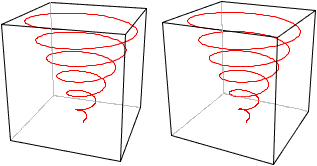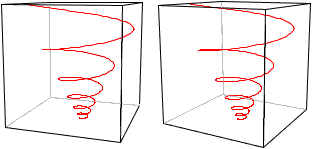Loxodrome, Spherical Helix
 ...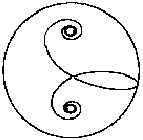... The loxodrome is a curve on the sphere, which cuts the meridians at a constant angle. They appear on the Mercator projection as straight lines. The parametric representation is  x=cos(t) cos [tan-1(at)] y=sin(t) cos[tan-1(at)] z= -sin [tan-1(at)]      (a is constant) You can find out x²+y²+z²=1. This equation means that the loxodrome is lying on the sphere.
Generally there is a loxodrome at every solid made by rotation about an axis.

Making of Spirals top
 ...... A strip of paper becomes a spiral, if you pull the strip between the thumb and the edge of a knife, pressing hard. The spiral becomes a curl where gravity is present.
You use this effect to decorate the ends of synthetic materials, such as the narrow colourful strips or ribbons used in gift-wrapping.
I suppose that you have to explain this effect in the same way as a bimetallic bar. You create a bimetallic bar by glueing together two strips, each made of a different metal. Once this bimetallic bar is heated, one metal strip expands more than the other causing the bar to bend.
The reason that the strip of paper bends is not so much to do with the difference in temperature between the top and bottom side. The knife changes the structure of the surface of the paper. This side becomes 'shorter'.
Incidentally, a strip of paper will bend slightly if you hold it in the heat of a candle flame.

 ...... Forming curls reminds me of an old children's game: Take a dandelion flower and cut the stem into two or four strips, keeping the head intact. If you place the flower into some water, so that the head floats on the surface, the strips of the stem will curl up. (Mind the spots.) A possible explanation: Perhaps the different absorption of water on each side of the strips causes them to curl up.

Mandelbrot Set Spirals top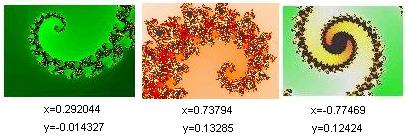The coordinates belong to the centre of the pictures.

You also find nice spirals as Julia Sets. Here is an example: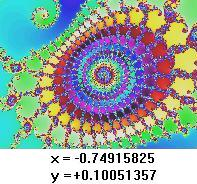You find more about these graphics on my page Mandelbrot Set.

You find nice spirals as a decoration of barred windows, fences, gates or doors. You can see them everywhere, if you are look around.
 ...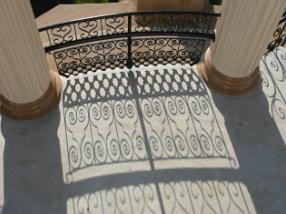... I found spirals worth to show at New Ulm, Minnesota, USA.  Americans with German ancestry built a copy of the Herman monument near Detmold/Germany in about 1900.  Iron railings with many spirals decorate the stairs (photo).  More about the American and German Herman on Wikipedia-pages (URL below)

Costume jewelleries also take spirals as motive.
 ...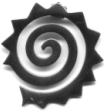... Annette's spiralSpirals, Spirals, Spirals top
Ammonites, antlers of wild sheep, Archimedes' water spiral, area of high or low pressure, arrangement of the sunflower cores, @, bimetal thermometer, bishop staff, Brittany sign, circles of a sea-eagle, climbs, clockwise rotating lactic acid, clouds of smoke, coil, coil spring, corkscrew, creepers (plants), curl, depression in meteorology, disc of Festós, double filament of the bulb, double helix of the DNA, double spiral, electron rays in the magnetic longitudinal field, electrons in cyclotron, Exner spiral, finger mark,  fir cone, glider ascending, groove of a record, head of the music instrument violin, heating wire inside a hotplate, heat spiral, herb spiral, inflation spiral,  intestine of a tadpole, knowledge spiral, licorice snail, life spiral, Lorenz attractor, minaret at Samarra (Iraq), music instrument horn, pendulum body of the Galilei pendulum, relief strip of the Trajan's column at Rome or the Bernward column at Hildesheim, poppy snail, road of a cone mountain, role (wire, thread, cable, hose, tape measure, paper, bandage), screw threads, simple pendulum with friction, snake in resting position, snake of Aesculapius, snail of the interior ear, scrolls, screw alga, snail-shell, spider net, spiral exercise book, spiral nebula, spiral staircase (e.g. the two spiral stairs in the glass dome of the Reichstag in Berlin),  Spirallala ;-), Spirelli noodles, Spirills (e.g. Cholera bacillus), springs of a mattress, suction trunk (lower jaw) of the cabbage white butterfly, tail of the sea-horse, taps of conifers, tongue and tail of the chamaeleon, traces on CD or DVD, treble clef, tusks of giants, viruses, volute, watch spring and balance spring of mechanical clocks, whirlpool, whirlwind.

Spirals on the Internet top

German

Jürgen Berkemeier
Fibonacci-Spiralen

Matheprisma
Bewegungsfunktionen (Spiralen 1 ) - (Spiralen online zeichnen)

Michael Komma
Fresnel-Beugung am Einzelspalt (Cornu-Spirale)

English

Ayhan Kursat ERBAS
Equiangular Spiral

Bob Allanson
This is a logarithmic spiral

David Eppstein  (Geometry Junkyard)

Eric W. Weisstein (MathWorld)
Spirals
Archimedean SpiralCircle InvoluteConical SpiralCornu SpiralCurlicue FractalFermat's SpiralHelix, Hyperbolic SpiralLogarithmic SpiralMice ProblemNielsen's SpiralPolygonal SpiralPrime SpiralRational SpiralSeashell, Spherical Spiral

Hop David (Hop's Gallery)
Riemann sphere, Ram's Horn, Spiral Tile

Jan Wassenaar
spiral

Keith Devlin
The Double Helix

Mark Newbold
Counter-Rotating Spirals Illusion

Richard Parris (Freeware-Programs)
winplot

French

Robert FERRÉOL (COURBES 2D )
SPIRALE
COURBES 3D (SPHÉRO-CYLINDRIQUE, SPIRALE CONIQUE DE PAPPUS, SPIRALE CONIQUE DE PIRONDINI, SPIRALE SPHÉRIQUE)

References   top
(1) Martin Gardener: Unsere gespiegelte Welt, Ullstein, Berlin, 1982 [ISBN 3-550-07709-2]
(2) Rainer und Patrick Gaitzsch: Computer-Lösungen für Schule und Studium, Band 2, Landsberg am Lech, 1985
(3) Jan Gullberg: Mathematics - From the Birth of Numbers, New York / London (1997) [ISBN 0-393-04002-X]
(4) Khristo N. Boyadzhiev: Spirals and Conchospirals in the Flight of Insects, The College Mathematics Journal,
Vol.30, No.1 (Jan.,1999) pp.23-31
(5) Jill Purce: the mystic spiral - Journey of the Soul, Thames and Hudson, 1972, reprinted 1992

Feedback: Email address on my main page